# Graham's number facts for kids

Kids Encyclopedia Facts

Graham's number is a very big natural number that was defined by a man named Ronald Graham. Graham was solving a problem in an area of mathematics called Ramsey theory. He proved that the answer to his problem was smaller than Graham's number.

Graham's number is one of the biggest numbers ever used in a mathematical proof. Even if every digit in Graham's number were written in the tiniest writing possible, it would still be too big to fit in the observable universe.

## Context

Ramsey theory is an area of mathematics that asks questions like the following:

Suppose we draw some number of points, and connect every pair of points by a line. Some lines are blue and some are red. Can we always find 3 points for which the 3 lines connecting them are all the same color?

It turns out that for this simple problem, the answer is "yes" when we have 6 or more points, no matter how the lines are colored. But when we have 5 points or fewer, we can color the lines so that the answer is "no".

Graham's number comes from a variation on this question.

Once again, say we have some points, but now they are the corners of an n-dimensional hypercube. They are still all connected by blue and red lines. For any 4 points, there are 6 lines connecting them. Can we find 4 points that all lie on one plane, and the 6 lines connecting them are all the same color?

By asking that the 4 points lie on a plane, we have made the problem much harder. We would like to know: for what values of n is the answer "no" (for some way of coloring the lines), and for what values of n is it "yes" (for all ways of coloring the lines)? But this problem has not been completely solved yet.

In 1971, Ronald Graham and B. L. Rothschild found a partial answer to this problem. They showed that for n=6, the answer is "no". But when n is very large, as large as Graham's number or larger, the answer is "yes".

One of the reasons this partial answer is important is that it means that the answer is eventually "yes" for at least some large n. Before 1971, we didn't know even that much.

## Definition

Graham's number is not only too big to write down all of its digits, it is too big even to write in scientific notation. In order to be able to write it down, we have to use Knuth's up-arrow notation.

We will write down a sequence of numbers that we will call g1, g2, g3, and so on. Each one will be used in an equation to find the next. g64 is Graham's number.

First, here are some examples of up-arrows:

•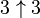$3\uparrow3$ is 3x3x3 which equals 27. An arrow between two numbers just means the first number multiplied by itself the second number of times.
• One can think of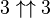$3 \uparrow \uparrow 3$ as$3 \uparrow (3 \uparrow 3)$ because two arrows between numbers A and B just means A written down a B number of times with an arrow in between each A. Because we know what single arrows are,$3\uparrow(3\uparrow3)$ is 3 multiplied by itself$3\uparrow3$ times and we know$3\uparrow3$ is twenty-seven. So$3\uparrow\uparrow3$ is 3x3x3x3x....x3x3, in total 27 times. That equals 7,625,597,484,987.
•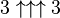$3 \uparrow \uparrow \uparrow 3$ is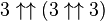$3 \uparrow \uparrow (3 \uparrow \uparrow 3)$ and we know$3\uparrow\uparrow3$ is 7,625,597,484,987. So$3\uparrow\uparrow(3\uparrow\uparrow3)$ is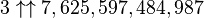$3\uparrow \uparrow 7,625,597,484,987$. That can also be written as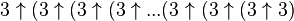$3\uparrow(3\uparrow(3\uparrow(3\uparrow . . .(3\uparrow(3\uparrow(3\uparrow3)$ with a total of 7,625,597,484,987 3s. This number is so huge, its digits, even written very small, could fill up the observable universe and beyond.
• Although this number may already be beyond comprehension, this is barely the start of this giant number.
• The next step like this is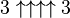$3 \uparrow \uparrow \uparrow \uparrow 3$ or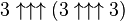$3 \uparrow \uparrow \uparrow (3 \uparrow \uparrow \uparrow 3)$. This is the number we will call g1.

After that, g2 is equal to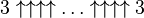$3\uparrow \uparrow \uparrow \uparrow \ldots \uparrow \uparrow \uparrow \uparrow 3$; the number of arrows in this number is g1.

g3 is equal to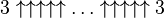$3\uparrow \uparrow \uparrow \uparrow \uparrow \ldots \uparrow \uparrow \uparrow \uparrow \uparrow 3$, where the number of arrows is g2.

We keep going in this way. We stop when we define g64 to be$3\uparrow \uparrow \uparrow \uparrow \uparrow \ldots \uparrow \uparrow \uparrow \uparrow \uparrow 3$, where the number of arrows is g63.

This is Graham's number.

## Related pagesGraham's number Facts for Kids. Kiddle Encyclopedia.